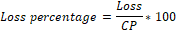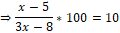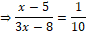Question of The Day28-03-2022

A Fruit seller incurred loss equal to the selling price of (x - 5) mangoes by selling (2x - 3) mangoes. If the loss percentage is equal to 10%. Find the value of x.

Correct Answer : a ) 6

Explanation :

According to the question,

Let the Selling price of 1 Mango be SP and the Cost price of 1 Mango be CP

Loss = Selling Price of (x - 5) mangoes = (x - 5) * SP

Selling price of (2x - 3) Mangoes = (2x - 3) * SP

We know that

Cost price of (2x - 3) mangoes = Selling price + Loss = (2x – 3 + x – 5) * SP = (3x – 8) * SP

⇒ (2x - 3)*CP = (2x – 3 + x – 5) * SP

⇒ (2x - 3)*CP = (3x – 8) * SP⇒ 10x – 50 = 3x – 8

⇒ 7x = 42

⇒ x = 6.

Hence, (a) is the correct answer.0